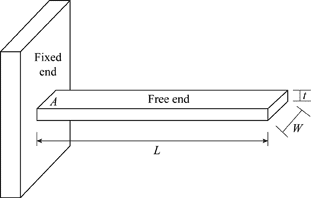# Torque on a Beam Due to Gravity

cs44167
Homework Statement:
A rigid beam of mass 7.78 kg and length 1.44 m is fixed at point P, around which the beam rotated (P is at the far leftmost point of the beam). What is torque on the beam due to gravity only?
Relevant Equations:
Torque = r*F*sin (theta)
w = mg
I tried using r * f * sin theta and calculated this:

1.14 m * 9.80 m/s/s * 7.78 kg = 109.7 N*m

this was wrong; I needed three significant figures so I did 1.10E2 N*m which was also wrong.

Since the torque is due to gravity; would it be -1.10E2 N*m since it’s angle is -270° which is -1?

Mentor
Homework Statement:: A rigid beam of mass 7.78 kg and length 1.44 m is fixed at point P, around which the beam rotated (P is at the far leftmost point of the beam). What is torque on the beam due to gravity only?
Homework Equations:: Torque = r*F*sin (theta)
w = mg

I tried using r * f * sin theta and calculated this:

1.14 m * 9.80 m/s/s * 7.78 kg = 109.7 N*m

this was wrong; I needed three significant figures so I did 1.10E2 N*m which was also wrong.

Since the torque is due to gravity; would it be -1.10E2 N*m since it’s angle is -270° which is -1?
Where did 1.14m come from in your calculation? A typo of 1.44m? And the force acts at the center of mass for the beam, not at the end of it...•PhanthomJay
cs44167
Where did 1.14m come from in your calculation? A typo of 1.44m? And the force acts at the center of mass for the beam, not at the end of it...Yes, the 1.14 was a typo - it should’ve been 1.44 which I had in my calculations.

What does it mean for the force to act at the center? Would that divide the radius into halves?

Mentor
Yes. It's hard to be sure without seeing the figure that has the problem, but if it's a simple horizontal cantilever beam supported only at the left side, and the beam is uniform density, then its COM is halfway out.

•PhanthomJay
Mentor
Is it like this?

https://media.cheggcdn.com/study/c0c/c0c63894-f03d-4021-9d86-9b6660f0bd54/DC-785V1.png•